# CBSEtips.in

## Thursday, 25 February 2021

### CBSE Class 7 Maths - MCQ and Online Tests - Unit 14 - Symmetry

#### CBSE Class 7 Maths – MCQ and Online Tests – Unit 14 – Symmetry

Every year CBSE students attend Annual Assessment exams for 6,7,8,9,11th standards. These exams are very competitive to all the students. So our website provides online tests for all the 6,7,8,9,11th standards’ subjects. These tests are also very effective and useful for those who preparing for any competitive exams like Olympiad etc. It can boost their preparation level and confidence level by attempting these chapter wise online tests.

These online tests are based on latest CBSE syllabus. While attempting these, our students can identify their weak lessons and continuously practice those lessons for attaining high marks. It also helps to revise the NCERT textbooks thoroughly

#### CBSE Class 7 Maths – MCQ and Online Tests – Unit 14 – Symmetry

Question 1.
How many lines of symmetry are there in the following figure?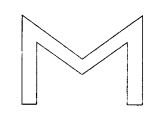(a) 4
(b) 3
(c) 2
(d) 1

Question 2.
How many lines of symmetry are there in the following figure?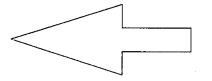(a) 1
(b) 2
(c) 3
(d) 4

Question 3.
How many lines of symmetry are there in the following figure?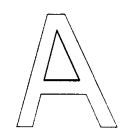(a) 2
(b) 1
(c) 4
(d) 3

Question 4.
How many lines of symmetry are there in a rectangle?
(a) 1
(b) 2
(c) 3
(d) 4

Question 5.
How many lines of symmetry are there in a regular pentagon?
(a) 1
(b) 2
(c) 3
(d) 5

Question 6.
How many lines of symmetry are there in the following figure?(a) 1
(b) 2
(c) 3
(d) 4

Question 7.
How many lines of symmetry are there in an equilateral triangle?
(a) 1
(b) 2
(c) 3
(d) 4

Question 8.
How many lines of symmetry are there in a square?
(a) 1
(b) 2
(c) 3
(d) 4

Question 9.
How many lines of symmetry are there in the following figure?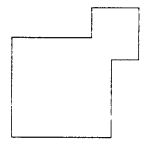(a) 1
(b) 2
(c) 3
(d) 4

Question 10.
How many lines of symmetry are there in a scalene triangle?
(a) 1
(b) 0
(c) 2
(d) 4

Question 11.
How many lines of symmetry are there in a rhombus?
(a) 1
(b) 2
(c) 3
(d) 4

Question 12.
How many lines of symmetry are there in following figure?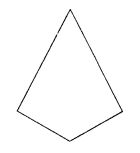(a) 1
(b) 2
(c) 3
(d) None of these

Question 13.
How many lines of symmetry are there in the following figure?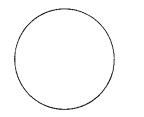(a) 1
(b) 2
(c) 3
(d) Infinitely many

Answer: (d) Infinitely many

Question 14.
How many lines of symmetry are there in an isosceles triangle?
(a) 4
(b) 3
(c) 1
(d) 2

Question 15.
Which of the following letters of English alphabet has reflectional symmetry about a vertical mirror?
(a) H
(b) J
(c) Z
(d) P

Question 16.
Which of the following letters of English alphabet has reflectional symmetry about a horizontal mirror?
(a) H
(b) K
(c) M
(d) W

Question 17.
The order of the rotational symmetry of the following figure about the point marked x is(a) 2
(b) 3
(c) 4
(d) 1

Question 18.
How many lines of symmetry are there in a parallelogram?
(a) 0
(b) 1
(c) 2
(d) None of these

Question 19.
How many lines of symmetry are there in a quadrilateral?
(a) 0
(b) 2
(c) 4
(d) None of these

Question 20.
The order of rotational symmetry of an equilateral triangle is
(a) 1
(b) 2
(c) 3
(d) 4

Question 21.
The order of rotational symmetry of a square is
(a) 1
(b) 2
(c) 3
(d) 4

Question 22.
What is the order of the rotational symmetry of the following figure?(a) 4
(b) 3
(c) 2
(d) 1

Question 23.
The order of the rotational symmetry of the following figure about the point marked x (cross) is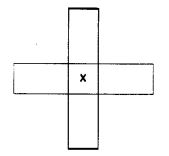(a) 1
(b) 2
(c) 3
(d) 4

Question 24.
The order of the rotational symmetry of the following figure about the point marked x is(a) 1
(b) 2
(c) 3
(d) 4

Question 25.
How many lines of symmetry are there in a regular hexagon?
(a) 2
(b) 4
(c) 6
(d) 3